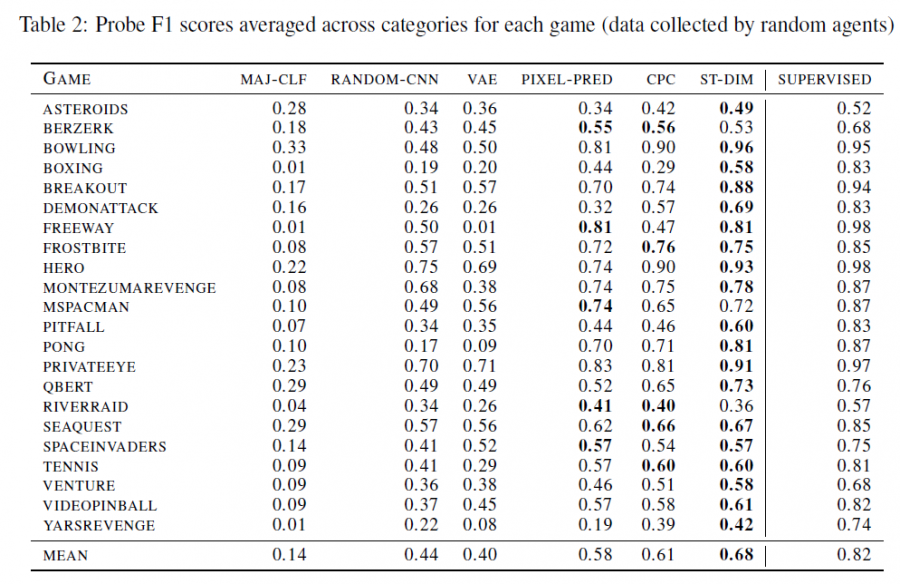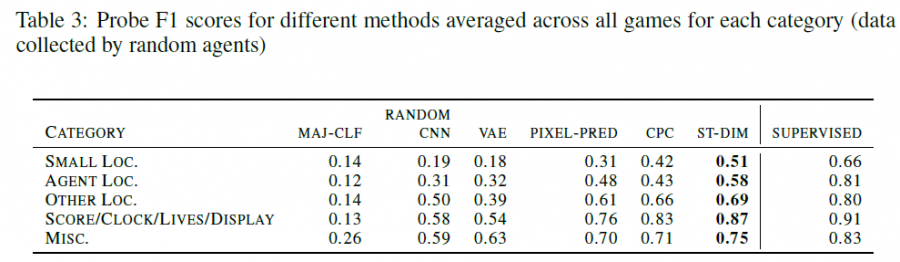# 单智能体强化学习算法

## Unsupervised State Representation Learning in Atari

Posted by MY on August 20, 2020

## 一、问题

RL中通常通过使用来自奖励的信号通过端到端学习来学习状态的表征，但是这种方法通常效率很低，而且学到的表征可能包含很多对RL决策不产生影响的信息。本文借鉴自监督学习中的对比学习方法来构建Atari像素游戏的状态表征。

## 二、解法### 2.2 loss函数的选择

#### 2.2.1 global-local objective

$\mathcal{L}_{G L}=\sum_{m=1}^{M} \sum_{n=1}^{N}-\log \frac{\exp \left(g_{m, n}\left(x_{t}, x_{t+1}\right)\right)}{\sum_{x_{i}^{*} \in X_{n e x t}} \exp \left(g_{m, n}\left(x_{t}, x_{t^{*}}\right)\right)}$

#### 2.2.2 local-local objective

$\mathcal{L}_{L L}=\sum_{m=1}^{M} \sum_{n=1}^{N}-\log \frac{\exp \left(f_{m, n}\left(x_{t}, x_{t+1}\right)\right)}{\sum_{x_{t}^{*} \in X_{n e x t}} \exp \left(f_{m, n}\left(x_{t}, x_{t^{*}}\right)\right)}$

## 三、实验内容## 五、优点

• 首个将Contrastive Learning引入RL中进行state representation的工作
• 相比于用CNN处理的像素画面，这种做法可以更多地提取有用的抽象信息，忽略无关的像素级别的细节

##### Share